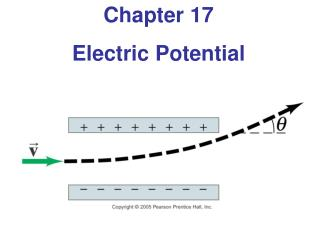# Chapter 17 Electric Potential - PowerPoint PPT PresentationDownload PresentationChapter 17 Electric Potential

Presentation Description
Download Presentation## Chapter 17 Electric Potential

- - - - - - - - - - - - - - - - - - - - - - - - - - - E N D - - - - - - - - - - - - - - - - - - - - - - - - - - -
##### Presentation Transcript

1. Chapter 17 Electric Potential

2. Units of Chapter 17 • Electric Potential Energy and Potential Difference • Relation between Electric Potential and Electric Field • Equipotential Lines • The Electron Volt, a Unit of Energy • Electric Potential Due to Point Charges

3. Units of Chapter 17 • Capacitance • Dielectrics • Storage of Electric Energy

4. 17.1 Electrostatic Potential Energy and Potential Difference The electrostatic force is conservative – potential energy can be defined Change in electric potential energy is negative of work done by electric force: (17-1)

5. 17.1 Electrostatic Potential Energy and Potential Difference Electric potential is defined as potential energy per unit charge: (17-2a) Unit of electric potential: the volt (V). 1 V = I J/C.

6. 17.1 Electrostatic Potential Energy and Potential Difference Only changes in potential can be measured, allowing free assignment of V = 0. (17-2b)

7. 17.1 Electrostatic Potential Energy and Potential Difference Analogy between gravitational and electrical potential energy:

8. 17.2 Relation between Electric Potential and Electric Field Work is charge multiplied by potential: Work is also force multiplied by distance:

9. 17.2 Relation between Electric Potential and Electric Field Solving for the field, (17-4b) If the field is not uniform, it can be calculated at multiple points:

10. 17.3 Equipotential Lines An equipotential is a line or surface over which the potential is constant. Electric field lines are perpendicular to equipotentials. The surface of a conductor is an equipotential.

11. 17.3 Equipotential Lines

12. 17.4 The Electron Volt, a Unit of Energy One electron volt (eV) is the energy gained by an electron moving through a potential difference of one volt.

13. 17.5 Electric Potential Due to Point Charges The electric potential due to a point charge can be derived using calculus. (17-5)

14. 17.5 Electric Potential Due to Point Charges These plots show the potential due to (a) positive and (b) negative charge.

15. 17.5 Electric Potential Due to Point Charges Using potentials instead of fields can make solving problems much easier – potential is a scalar quantity, whereas the field is a vector.

16. 17.7 Capacitance A capacitor consists of two conductors that are close but not touching. A capacitor has the ability to store electric charge.

17. 17.7 Capacitance Parallel-plate capacitor connected to battery. (b) is a circuit diagram.

18. 17.7 Capacitance When a capacitor is connected to a battery, the charge on its plates is proportional to the voltage: (17-7) The quantity C is called the capacitance. Unit of capacitance: the farad (F) 1 F = 1 C/V

19. 17.7 Capacitance The capacitance does not depend on the voltage; it is a function of the geometry and materials of the capacitor. For a parallel-plate capacitor: (17-8)

20. 17.8 Dielectrics A dielectric is an insulator, and is characterized by a dielectric constant K. Capacitance of a parallel-plate capacitor filled with dielectric: (17-9)

21. 17.8 Dielectrics Dielectric strength is the maximum field a dielectric can experience without breaking down.

22. 17.8 Dielectrics The molecules in a dielectric tend to become oriented in a way that reduces the external field.

23. 17.8 Dielectrics This means that the electric field within the dielectric is less than it would be in air, allowing more charge to be stored for the same potential.

24. 17.9 Storage of Electric Energy A charged capacitor stores electric energy; the energy stored is equal to the work done to charge the capacitor. (17-10)

25. 17.9 Storage of Electric Energy The energy density, defined as the energy per unit volume, is the same no matter the origin of the electric field: (17-11) The sudden discharge of electric energy can be harmful or fatal. Capacitors can retain their charge indefinitely even when disconnected from a voltage source – be careful!

26. 17.9 Storage of Electric Energy Heart defibrillators use electric discharge to “jump-start” the heart, and can save lives.

27. Summary of Chapter 17 • Electric potential energy: • Electric potential difference: work done to move charge from one point to another • Relationship between potential difference and field:

28. Summary of Chapter 17 • Equipotential: line or surface along which potential is the same • Electric potential of a point charge:

29. Summary of Chapter 17 • Capacitor: nontouching conductors carrying equal and opposite charge • Capacitance: • Capacitance of a parallel-plate capacitor:

30. Summary of Chapter 17 • A dielectric is an insulator • Dielectric constant gives ratio of total field to external field • Energy density in electric field: